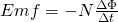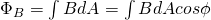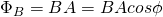# Physics - Electromagnetism - Electromagnetic Induction and Faraday's lawImage source:## Introduction

Hello it's a me again Drifter Programming! Today we continue with Electromagnetism to get into Electromagnetic Induction and also Faraday's law. I'm back from Vacation and so back again into writing science related articles :) The previous week was wonderful! I needed this pause of "thinking" so badly...So, without further do, let's get finally started with today's topic!

## Electromagnetic Induction

In Definition, Electromagnetic Induction is the production of voltage or electromotive force due to a changing magnetic field. It's the process of generating current with a magnetic field and only occurs when the magnetic field and electric condcutor have a relative motion to one another. It was discovered by Michael Faraday in the 1830s and his law is still used in many electrical applications today (we will get into this law later on during this article...). The two most important devices that depend on electromagnetic induction are: electric generators and electric transformers, which play critical roles in producing and regulating electric current in our daily lives.

Electromagnetic induction is generated in two ways:

1. When a electric conductor is kept in a moving magnetic field AND
2. When a electric conductor is constantly moving within a static magnetic field

You can clearly see that motion is of great importance. The whole phenomenon is independent of which of the two is moving. The conductor and magnetic field just need to have a relative velocity/motion to each other. Faraday discovered this phenomenon by moving a bar magnet through an electric coil. He noticed a voltage chage in the circuit and deduced the factors that influenced the electromagnetic induction.

The factors that this electromagnetic induction depended on where:

• number of coils
• strength of the magnet
• the changing magnetic field
• the speed of the relative motion between the coil & magnet

## Experiments

The procedure that I described and that Faraday used is of course an experiment. It's one of the experiments with which we can study/test electromagnetic induction.

### Coil of wire

Let's consider a coil of wire connected to a galvanometer. When the magnet is stationary the meter is showing no current. When moving the magnet either towards or away from the coil the meter of course shows current, which is of course caused by electromagnetic induction.

### Solenoid with D.C supply

By replacing the magnet with a solenoid connected to a D.C power supply current flows through the solenoid. As we know from the previous posts, such a solenoid is a magnetic field source/generator. When keeping te solenoid stanionary there is no current in the coil of wire. There is current only when the solenoid and coil of wire are moving relative to each other, which causes electromagnetic induction.

### Circuit of before with switch

By introducing a switch into the circuit of before and by also keeping both the solenoid and coil of wire stationary relative to each other. There is induced current in the coil of wire only when the switch is turned on and off, which means that the current in the solenoid is changing (changing magnetic field).

In all those experiments common element is the changing magnetic flux. From all that we can see that induced current is produced by changing magnetic fields, which have changing magnetic flux.

The following link describes an experiment that you can try at home:

http://tap.iop.org/fields/electromagnetism/414/page_46948.html

As we described till now there is a relationship between the electrical voltage and changing magnetic field. Michael Faraday stated:

"That a voltage is induced in a circuit whenever relative motion exists between a conductor and a magnetic field and that the magnitude of this voltage is proportional to the rate of change of the flux”

We previously already noted the factors that determine the induced voltage. Let's get into each one more in-depth now:

• When increasing the number of coils we also increase the induced voltage (proportional) by the same amount
• When increasing the relative speed/motion between the coil and magnet we also increase the induced emf produced (proportional) by the same amount
• When increasing the strength of the magnetic field we also increase the induced voltage (proportional)

The equation is described like this:where:

• N is the number of coils
• Φ is the magnetic flux
• The voltage is noted as ε or Emf from the term "ElectroMotive Force"

Magnetic flux was defined as:where:

• B is the magnetic field strength
• A the area
• φ the angle between the magnetic field lines and normal vector of the surface

When having a uniform magnetic field and "simple" surface we have:More things about Electromotive force will come next time...

## REFERENCES:

Mathematical equations that I had in this post where drawn using quicklatex

### Electric fields:

Getting into Electromagnetism -> electromagnetim, electric charge, conductors, insulators, quantization

Coulomb's law with examples -> Coulomb's law, superposition principle, Coulomb constant, how to solve problems, examples

Electric fields and field lines -> Electric fields, Solving problems around Electric fields and field lines

Electric dipoles -> Electric dipole, torque, potential and field

Electric charge and field Exercises -> examples in electric charges and fields

### Electric flux:

Electric flux and Gauss's law -> Electric flux, Gauss's law

Applications of Gauss's law (part 1) -> applying Gauss's law, Gauss applications

Applications of Gauss's law (part 2) -> more Gauss applications

Electric flux exercises -> examples in electric flux and Gauss's law

### Electric potential:

Electric potential energy -> explanation of work-energy, electric potential energy

Calculating electric potentials -> more stuff about potential energy, potential, calculating potentials

Millikan's Oil Drop Experiment -> Millikan's experiment, electronvolt

Cathode ray tubes explained using electric potential -> cathode ray tube explanation

Electric potential exercises (part 1) -> applications of potential

Electric potential exercises (part 2) -> applications of potential gradient, advanced examples

### Capacitance:

Capacitors (Condensers) and Capacitance -> Capacitors, capacitance, calculating capacitance

How to solve problems around Capacitors -> combination, solving problems, simple example

Electric field energy and density -> Electric field energy, energy density

Dielectric materials -> Dielectrics, dielectric constant, permittivity and strength, how to solve problems

Electric capacitance exercises -> examples in capacitance, energy density and dielectrics

### Current, resistance and EMF:

Electric current -> Electric current, current density

Electrical resistivity and conductivity -> Electrical resistivity, conductivity, thermal coefficient of resistivity, hyperconductivity

Electric resistance -> Resistance, temperature, resistors

Electromotive Force (EMF) and Internal resistance -> Electromotive force, internal resistance

Power and Wattage of Electronic Circuits -> Power in general, power/wattage of electronic circuits

Electric current, resistance and emf exercises -> exampes in all those topics

### Direct current (DC) circuits:

Resistor Combinations -> Resistor combinations, how to solve problems

Kirchhoff's laws with applications -> Kirchhoff's laws, how to solve problems, applications

Electrical measuring instruments -> what are they?, types list, getting into some of them, an application

Electronic circuits with resistors and capacitors (R-C) -> R-C Circuit, charging, time constant, discharging, how to apply

RC circuit exercises -> examples in Kirchhoff, charging, discharging capacitor with/without internal resistance

### Magnetic field and forces:

Magnetic fields -> Magnetism, Magnetic field

Magnetic field lines and Gauss's law of Magnetism -> magnetic field lines, mono- and dipoles, Flux, Gauss's law of magnetism

The motion of charged particles inside of a magnetic field -> straight-line, spiral and helical particle motion

Applications of charged particle motion -> CERN, Cyclotrons, Synchrotrons, Cavity Magetron, Mass Spectrometry and Magnetic lens

Magnetic force applied on Current-Carrying Conductors -> magnetic force on current-carrying conductors/wires, proofs

Magnetic force and torque applied on current loops (circuits) -> magnetic force on current loops, magnetic moment and torque

Explaining the Physics behind Electromotors -> tesla, history and explaining the physics behind them

Magnetic field exercises -> examples in magnetic force, magnetic flux, particle motion and forces/torque on current-carrying conductors

### Magnetic field sources:

Magnetic field of a moving charged particle -> moving charge, magnetic field, force between parallel charged particles

Magnetic field of current-carrying conductors -> magnetic field of current, Biot-Savart law

Force between parallel conductors and the magnetic field of a current loop-> force between parallel conductors, magnetic field of current loop

Ampere's law and Applications -> Ampere's law, applications

Magnetic materials -> Magnetic materials, classification and types, material examples

Displacement current -> Displacement current, Extension of Ampere's law

Exercises in Magnetic field sources -> examples all around magnetic field sources

And this is actually it for today's post!

Next time in Physics we will get into Motional Electromotive Force...

Bye!

H2
H3
H4
3 columns
2 columns
1 column
Join the conversation now